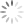Richtek

# Analysis of Buck Converter Efficiency

## Abstract

The synchronous buck circuit is wildly used to provide non-isolated power for low voltage and high current supply to system chip. To realize the power loss of synchronous buck converter and to improve efficiency is important for power designer. The application note introduces the analysis of buck converter efficiency and realizes major power component loss in synchronous buck converter.

## Buck converter power loss analysis

To realize the power loss in converters is important for converter design optimization. Figure1 shows the general single phase synchronous buck converter circuit. The major power losses in synchronous buck converter circuit are listed as bellow :

A : Power semiconductor loss

B : Inductor loss

C : Driver loss

D : PCB trace lossFigure 1. Synchronous buck converter

## Power loss calculation

Low-power loss and highly efficient synchronous buck converters are in great demand for advanced micro-processors. The application note introduces and provides how to calculate the majority of power losses in a typical synchronous buck converter occur in the following components based on that the converter works in continuous conduction mode (CCM) fixed switching frequency, fixed input voltage and fixed output voltage.

A : Power semiconductor loss :

HMOS (High-Side MOSFET) summarizes to include : switching on & off and conduction loss.

LMOS (Low-Side MOSFET) summarizes to include : conduction, dead-time and reverse recovery charge loss.

HMOS switching on loss :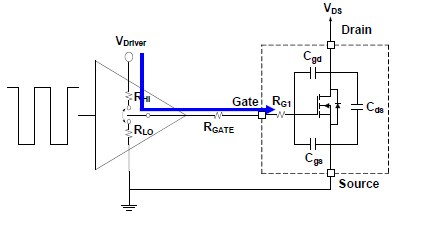Figure 2. HMOS Driver switching onFigure 3. HMOS switching on loss area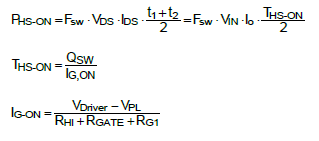HMOS conduction loss :

The conduction loss of high-side MOSFET is determined by the on-resistances of the MOSFET and the transistor RMS current.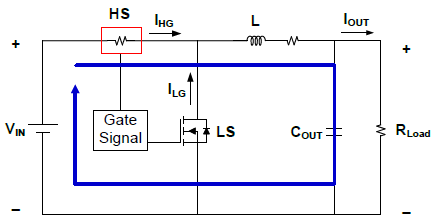Figure 4. HMOS conduction on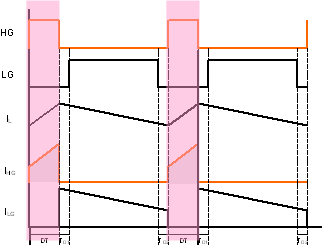Figure 5. HMOS conduction on period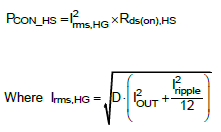LMOS conduction loss :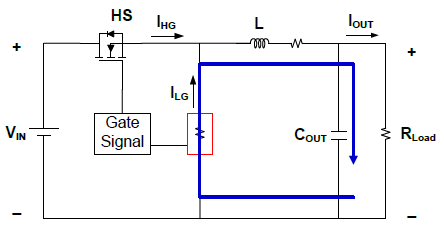Figure 6. LMOS conduction on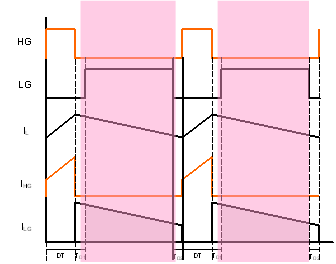Figure 7. LMOS conduction on periodLMOS dead time body diode loss :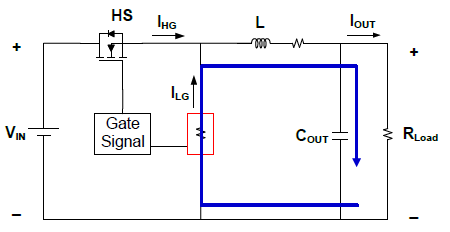Figure 8. LMOS body diode conduction on

Figure 9. LMOS body diode conduction on period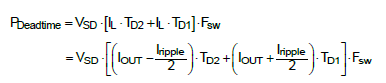LMOS reverse recovery charge loss :Figure 10. LMOS body diode reverse recovery periodB : Inductor DC & AC loss

Inductor DC loss :Figure 11. Current through inductor pathFigure 12. Inductor current path periodInductor core loss :

Inductor core losses are major caused by an alternating magnetic field in the core material. The losses are a function of the operating frequency and the total magnetic flux swing. The core loss may vary from one magnetic material to another.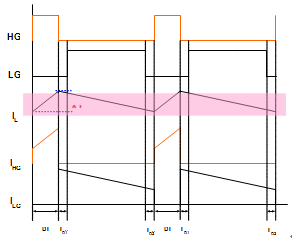Figure 13. Inductor ripple current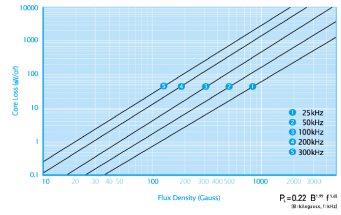Figure 14. Core loss curve

The calculated and/or measured core loss is often directly provided by the inductor supplier. If not, a formula can be used to calculate the core loss as bellow :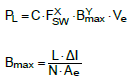The PL is the power loss (mW),

Fsw : operating frequency

B : peak flux desity in Gauss

Ve : effective core volume

The specific value of C, X and Y are core loss parameters for each material

C: Gate driver loss :

The gate driver loss is straightforward given by MOSFET driver to charge /discharge total HMOS and LMOS Qg. The gate driver loss is depending on MOSFET total gate charge, driver voltage and Fsw.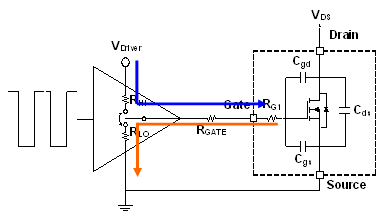Figure 15. Driver turns on and off pathFigure 16. MOSFET driver on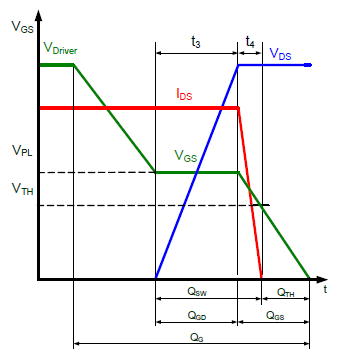Figure 17. MOSFET driver offD : PCB loss :

Figure 18 could be illustrated as Figure 19 and Figure 20 with Rtr1~Rtr7 with loop1 (HMOS conduction) and loop2 (LMOS conduction) in detail.Figure 18. PCB trace diagramFigure 19. PCB loop1 trace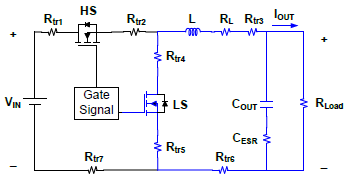Figure 20. PCB loop2 trace## Power loss measurement and calculation comparison

Although the buck converter power loss calculated equations are well introduced and documented. In order to check the accuracy of these power loss equations, Table1 shows the typical buck converter application parameter and Figure 21 illustrates the efficiency comparison between measurement and calculation.

Table 1. Buck converter application parameter

 IC RT8120 VIN 12V Vout 1.2V FSW 300kHz VDD 12V L 1mH DCR 1.2mW HMOS BSC090N03LS LMOS BSC090N03LS*2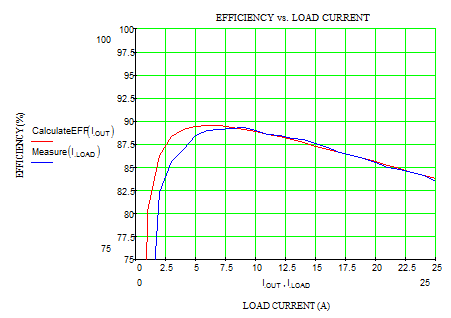Figure 21. Measurement and calculation of efficiency comparison

Figure 22 shows the key component loss in buck converter including HMOS, LMOS, inductor, driver and PCB trace loss. Readers can check what the major loss contributed in each system loading.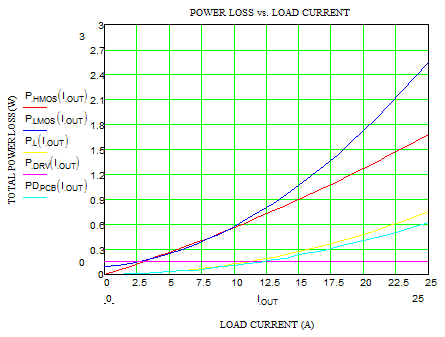Figure 22. Key component loss in buck converter

Figure 23 shows detail component loss in buck converter and illustrates the loss v.s Iout in the curve.

HMOS : PHSW (Switching loss) and PHCOD (Conduction loss)

LMOS : PLCOD (Conduction loss), PL_DIODE (Dead-time body diode loss) and PRR (Reverse recovery loss)

Inductor : PL (Inductor DC & core loss)

Driver : PDRV (Gate driver charge loss)

PCB : PPCB (PCB trace loss)Figure 23. Detail power loss in buck converter

## Conclusion

This application document analyzes power loss in synchronous buck converters and presents the detailed calculations for each part of the power loss. The loss calculation also compares with real buck converter measurement and provides the key component loss data to consider how to improve the buck converter efficiency for component and PCB plane consideration.

## Reference

 Leon Chen, “Power Loss Analysis for Synchronous Buck Converter”, Application Engineer Dept data, 2013.

 Nelson Garcia, “Determining Inductor Power Losses”, Coil craft Document 486, 2005.Table of Content